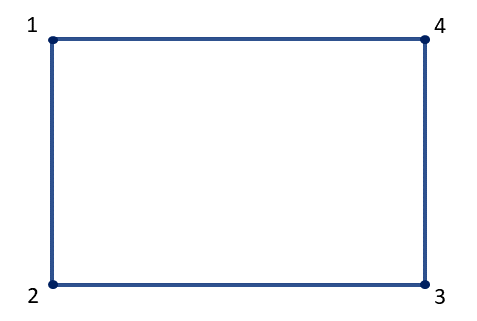# Problem 51820. Count unique orderings of vertices of a polygon

Cody Problem 2671 asks us to determine whether four points can be the corners of a rectangle. The points are not necessarily input in order (either clockwise or counterclockwise). My initial attempt at the problem involved determining how the points might be presented. If the corners are numbered as shown below, then they can be input in 24 ways.
However, for the rectangle problem, many of the 24 ways are essentially the same. For example, 2341, 3214, and 4123 are effectively the same as 1234 because the numbers of the corners could be shifted around the rectangle. In fact, only three of the 24 ways are different (1234, 1243, and 1324).
Write a function to determine the unique orderings of vertices of a polygon with n sides.### Solution Stats

100.0% Correct | 0.0% Incorrect
Last Solution submitted on Oct 03, 2023

### Community Treasure Hunt

Find the treasures in MATLAB Central and discover how the community can help you!

Start Hunting!